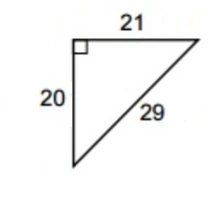# A Physics Derivation of Pythagorean Triples?

In the Chinese mathematical text Jiuzhang Suanshu, we find an interesting problem that requires the Pythagorean triples formula to solve.Chapter 9: Gougu Problem 14

[There are two persons standing at the same location. Person A moves at a speed of 7. Person B moves at a speed of 3. Person B moves east. Person A first moves 10 bu south, then diagonally northeast until he meets person B once more. How far did each man travel?]

• bu (步) literally means pace, a Chinese unit of length that measures roughly 1.6 meters long.

The units of the speed are not specified, but it is reasonable to assume that the speed is measured in bu per second, which measures 1.6 meters per second.

For calculating the sides a right triangle with integer sides, the solution prescribed by this 2000-year old text uses the formula

(a,b,c)=(1/2(m^2n^2)mn1/2(m^2+n^2)),

where m=7 and n=3 are the speeds of person A and person B respectively. This formula is indeed the formula for generating Pythagorean triples such that all sides of the right triangle are mutually coprime. There is no derivation provided in the Jiuzhang Suanshu, so I shall provide one using basic geometry and a little physics (which the ancient Chinese had to some degree).

For the full derivation, check out my wiki page here: https://math-physics-problems.wikia.com/wiki/Chinese_Derivation_of_Pythagorean_Triples

Advertisements
Categories: